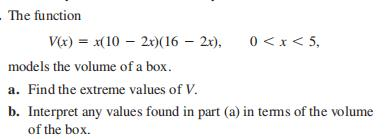### Still have math questions?

Trigonometry
QuestionThe function $$V ( x ) = x ( 10 - 2 x ) ( 16 - 2 x ) , 0 < x < 5$$ , models the volume of a box.

a. Find the extreme values of $$V$$ .

b. Interpret any values found in part (a) in tems of the volume of the box.

$$\left. \begin{array} { l } { \text { (a) Maximum value is } 144 \text { at } x = 2 \text { . } } \\ { \text { (b) The largest volume of the box is } 144 \text { cubic units, and it oc- } } \\ { \text { curs when } x = 2 \text { . } } \end{array} \right.$$